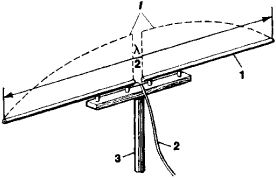# half-wave dipole

See dipole.

## Half-Wave Dipole

a radiator of electromagnetic waves that consists of a straight thin conductor or of a slot in the metal wall of a wave guide. The length of the conductor or slot is equal to approximately half the radiator’s operating wavelength. Half-wave dipoles are used, for example, as simple antennas for radio communication and as receiving antennas for television (Figure 1). They are also used as radiating elements of antenna arrays in communication and radar systems.

To the gap in the center of the dipole there is connected a feeder, or transmission line, which is either a balanced line or an unbalanced line with a balancing unit. The feeder connects theFigure 1. Simple television antenna: (1) half-wave dipole, (2) feeder, (3) support, (λ) operating wavelength; the broken line shows the distribution of the current / along the dipole

dipole to the transmitter or receiver. The radiation pattern of a half-wave dipole is a circle in a plane perpendicular to the axis of the dipole and a symmetrical figure eight in a plane containing the axis. The front-to-rear ratio of a half -wave dipole is 1.64. The radiation resistance is ~ 73 ohms.

## half-wave dipole

[′haf ¦wāv ′dī‚pōl]
(electromagnetism)
References in periodicals archive ?
Ideally, the half-wavelength slot should be complementary to the half-wave dipole. A slot ring dipole antenna is designed based on the most common and the most basic microstrip quarter-wave dipole.
The most common form of dipole antenna is the half-wave dipole, in which each of the two rod elements is approximately 1/4 wavelength long, so the whole antenna is a half-wavelength long.
Since the wire antenna is similar to a half-wave dipole and the cable in a typical setup usually much longer than the board size, the effective length of the wire antenna can be approximated as the cable length, [l.sub.cable].
Two half-wave dipole antennas 900 MHz and 1800 MHz were used as the exposure sources for this study.
Each of them can be simply model by a half-wave dipole connected to the different positions in feed line.
The first example considers a half-wave dipole placed directly on the lossy ground, Fig.2.
The large effect of directivity is easily seen when comparing a horn antenna with as much as 30- dBi gain--dBi means dB relative to isotropic--and a much less directional half-wave dipole with 2.14-dBi gain.
A half-wave dipole antenna is used as a standard of comparison.
A reflector antenna was used for signal transmission, and a shortened half-wave dipole antenna in the vertical position was used for signal reception.
A standard half-wave dipole antenna was used as the exposure source for this study.
In formula (2), the dipole element can be equivalent to a half-wave dipole and the array-element function can take cos[([pi]/2) sin[delta]]/cos[delta].

Site: Follow: Share:
Open / Close